Home

# Ideas for Propellantless Propulsion

It could easily be argued that the greatest challenge facing the promise of long-distance space travel is the aspect of propulsion.  When vehicles on Earth’s surface or within its waters or atmosphere move about, they generally do so by pushing against their surrounds.  Cars use wheels to push against the road, boats use propellers or paddles to push against the water, and helicopters use rotors to push against the air.

Vehicles in space don’t have that luxury.  With no surrounding mass to push against, the only way a spacecraft can move forward is by throwing material – usually rocket exhaust – in the opposing direction.  This all works very well, until that is, the material to be ejected is used up.  Thereafter the craft drifts at constant velocity and at the mercy of gravity .

Overcoming this limitation appears to leave us only two options: either bring along more material when starting a journey, or find a way of ejecting the material at higher speeds.  The first option is problematic because it makes the vehicle heavier: making it harder to launch against gravity in the first place, and harder to accelerate once in orbit.  The second option seems wiser and might be achieved by finding more powerful chemicals or higher-energy ‘ion thrusters’.

But for long journeys, both methods are doomed to failure.  Once the material is gone, it’s gone, and can’t be re-ejected.

What then if there were a way of producing forward motion without pushing against external objects or ejecting propellants?

This suggestion must sound crazy, impossible in fact.  Physics laws tell us that the interaction between two objects produces equal forces in opposite directions; such that two components of a spaceship pushing against each other could produce no net force in any direction.   This is often phrased as “for every action there is an equal and opposite reaction”.

This is Newton’s third law of motion and understood in terms of conservation of momentum.  It is what rockets rely on as they push a small amount of mass (the exhaust) backward at high speed, to move a large amount of mass (the rocket) forward at a lesser speed.  In fact it is what all forms of transport ultimately rely on: for a car to drive forward it needs to push the Earth slowly backward – momentum is conserved in both situations.

In an earlier article (see here), I argued that this law is just an approximation, albeit a very reliable one for most situations.  The reason is that the speed of light is finite, and a change in motion of one charged particle takes a short while to be communicated to the other.  As a result there is a delayed response and momentum can end up not being perfectly preserved.

I also suggested it may be possible to take advantage of this to accelerate a spacecraft without ejecting a propellant.  And that providing we had enough energy to power the process, the velocity would keep increasing essentially without limit.

This suggestion caught the attention of a few people (including a science fiction author) who were keen to know the practical aspects of this idea – about how it might be constructed, and whether the net force would be enough to be usable.  Here I’ll attempt to do just that, although the method will be different from the one earlier described.

#### The carousel

To understand the concept it is best to start with a simple analogy.

There is a carousel (a circular rotating platform, also called a merry-go-round) in a playground.  Two boys stand on it.  One stands in the middle and the other on the outside edge.  They each have a supply of tennis balls that they will throw at the other.  They throw balls at the same speed and simultaneously.  We’ll also say that when they throw a ball in a particular direction, they throw another ball in the opposite direction and at the same speed.  This is to prevent them from accelerating when throwing balls.  We’ll also say that they throw the balls once per second and at a speed of 5m/s.

Start with situation where the carousel is not rotating.  Each boy throws a ball toward his opponent while throwing another ball away/backward.  A short while later the balls arrive at their target and apply a force.  If the carousel’s surface was highly slippery, at the point when the balls were thrown, neither boy would move.  But when the balls hit, both boys would slide backward.  But we’ll say the surface isn’t slippery and they wear rubber shoes.  In this case the carousel floor would be stretched slightly.

Now to start the carousel rotating.  The boys throw their projectiles and now find they both miss – although for different reasons.  The one standing in the middle of the carousel misses because the other has moved from where he was when ball was thrown.  While the one standing on the outside misses because his sideways speed gets added to the ball, throwing it off-target.

Shortly afterward they each realise their mistake and adjust their aims: the one in the middle aims at future position of the “outsider”, while the “outsider” aims slightly in the opposing direction of the carousel’s motion.

It might seem that everything is back to normal, and that the resulting forces of impact are equal and opposite.  But that’s not the case.  When the boy in the middle threw his ball, it hit the “outsider” at the same speed and applied the same outward force.  It also applied a force in the reverse direction of carousel motion – however we will be ignoring this for this exercise.  Now when the “outsider” threw his ball, he needed to do so at a backward angle, in order to negate the carousel’s motion.  As a result the speed of the ball in the direction of the target was reduced and the ball applied less force.  This diagram explains the situation: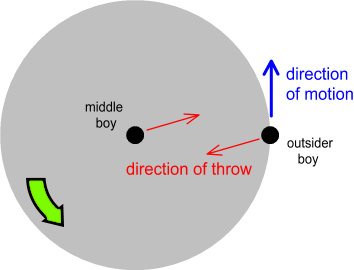From the perspective of someone standing on the carousel, the forces are no longer equal and opposite.  Instead there is a net force in the outward direction.

Now step this up a notch.  We increase the speed of the carousel until it is moving at the same speed as the boys throw the balls – 5m/s.  The boy in the middle can still hit the outsider by aiming at a future position even further ahead.  But the outsider finds he can no longer hit the one in the middle – even increasing the angle of throw to 90 degrees won’t do it.

The force is now one-sided and fully outward.  If the carousel were mounted on a slippery surface, we would expect it to launch slightly in that direction when the ball hit the “outsider” target.

#### Circling charges

Alas nothing useful has been achieved here because we are propelling the carousel by ejecting tennis balls in the opposing direction, making them an “external propellant”.  But this scenario was just an analogy to the main point that follows.

Let’s change the situation by considering two positively-charged spheres, such as what would found atop Van de Graaff generators.  They are connected by a joining rod.  One sits motionless while the other is made to orbit it.

As before, start with the situation where they are standing still (no orbiting).  Each sphere exerts a repulsive force of equal and opposite magnitude on the other, and the connecting rod is stretched, preventing them from moving apart.  As a result there is no net force and everything remains still.

Now start the orbiting process.  This can be done by placing them on a turntable with the motionless sphere in the centre.  To prevent the turntable wobbling, we’ll also place an uncharged sphere of identical mass and distance on the opposite side of the orbiting sphere.

Each sphere continues to push against the other but the situation is a bit different.  Referring back to the carousel example, the boys represent positive charges and the tennis balls represent a radiating electric field.

Just as with the boys on the carousel, the field that radiates from the orbiting sphere needs to originate from a slight angle in order to hit the motionless sphere.  This means it will hit the motionless sphere at a speed slightly less than c, the speed of light.  Whereas the field that comes off the motionless sphere will continue to hit the orbiting sphere at speed c.  Actually it will be slightly greater than c, due to the forward motion of the sphere being hit, but we’ll ignore this for now.  This diagram describes the situation: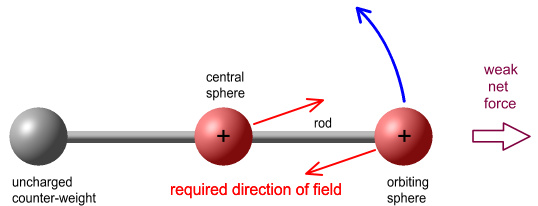To understand the implications of this, refer back to earlier chapters (here & here) where it argues that electrical force is a function of impacting field speed, such that (for like-charges) force will decrease as the field speed decreases, much like how the tennis ball’s force decreased with impact.  If this is correct, then it would seem that there would be a net force in the outward direction, toward the orbiting sphere.  If the turntable were sitting on a frictionless surface we would expect it to launch in that direction.

Unfortunately this is not helpful because the force direction is continually changing, meaning all that would happen is that the turntable would wobble.  So let’s make a couple of changes.

The first change is to replace the orbiting sphere with a charged ring that circles the central sphere (and we can remove the uncharged sphere on the opposite side).  The second is to raise the central sphere so that it is above the ring, but still on its axis of rotation.

This animation shows the result.  Here the whole system has been rotated 90 degrees.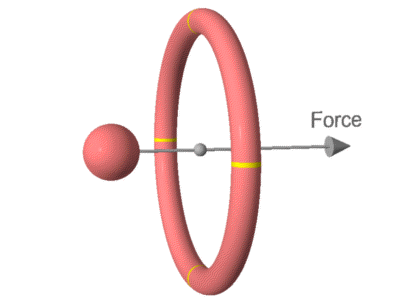Looking at the diagram, the electric field that emits from the rotating ring will need to originate at a slight angle (opposite to the direction of rotation) in order to hit the sphere.  As a result it will hit the sphere at a speed below c and exert less force.  The faster the rotation, the greater the angle will be required, and the less the impacting field speed and force.  Whereas the field that comes from the sphere will impact the ring at speed c, and exert full force.  As a result the forces will be unequal and there will be a net force toward the right, causing the system to accelerate in that direction.

To appreciate this better, imagine that the ring were rotating at the speed of light.  Obviously this can’t be done in practice!  But this is like the earlier example where the carousel was rotating at the same speed as the balls were thrown.  In this case none of the field originating from the ring will be able to hit the sphere, no matter what angle it emits from.  All the electric force will come from the sphere impacting on the ring, pushing the system to the right.

#### Doing the calculations

Now comes the interesting part: figuring out just how much force we are talking about.

There are actually two considerations involved.  The first is the reduced field-speed at impact, as discussed already.  The second is the spreading of field with time.

Going back to our original example, imagine that instead of tennis balls, the boys had toy shotguns.  These guns ejected a spray of (harmless) pellets that radiated from the end of each barrel in a uniform conical distribution.  The further away the target, the fewer pellets hit, and the less the force.  Also if the person firing the gun was moving backward at the time, the pellets would take longer to get there.  As a result, they would spread even wider and the force they applied would be weaker again.

The same principle applies to electric fields emitted from charges.  If the charge is moving away from the target, and both the source and target have the same charge sign (e.g. both positive), the target receives less force on account of: 1) the impacting field speed is reduced, and 2) the field has spread wider.

Experimenting with the sphere’s positioning tells us that the maximum force will be achieved when the height of the sphere above the ring is 1/squareroot(2) of the ring’s radius, i.e. 71% of the radius, putting it at a 35 degree elevation (this is what is the animation shows).

The calculation for the net force ends up being rather messy, containing many square roots and cubes.  But it can be simplified to a convenient ‘first order’ expression :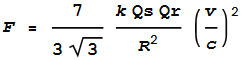--- (1)

Where:
F = net force
Qs = charge on sphere
Qr = charge on ring
R = large radius of ring
k = Coulomb’s constant
v = velocity of ring

So it would appear we need to: 1) maximise the charge on both the sphere and ring, 2) maximise the velocity, and 3) minimise the radius.

For a hypothetical setup, we’ll set the radius at 1 metre.  Next we need to make the charge as large as we can.  The sphere could be something that sits atop a Van de Graaff generator.  Looking up what a typical voltage would be on that, it’s about 450kV.  For a sphere of diameter 30cm, that translates to a charge of 7.5x10-6 C = 7.5 micro-coulombs .  Assume the ring was a hollow tube and also held the same charge.

We now have all the variables except velocity.  We want that to be as high as possible.  That means: make it as high as we can without having centrifugal force break the ring apart.  This velocity will depend on the strength and density of the material.  Surprisingly, it doesn’t depend on the large radius of the ring or its cross-sectional thickness.  The maximum velocity you can spin a ring at before it stretches beyond its damage point turns out to be given by this formula: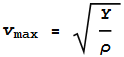--- (2)

where Y is the yield-strength of the material and ρ is the density.  A table on Wikipedia  shows the best conductive (metallic) candidate is a steel with yield-strength 2617MPa and density 8g/cm3.  This gives a maximum rotation speed of 570m/s.

Plugging those numbers in to our force equation gives us... 2.5x10-12 N

2.5 pico-Newtons!?  That’s nothing to get excited about and might not even be measureable, even in zero gravity.  Given that we are violating Newton’s force laws we shouldn’t expect anything that would be easily noticeable.

Still, let’s see what might be done to boost the results.  There are four variables to play with: two charges, radius, and velocity.

We could increase force by reducing radius.  By reducing the radius to 10cm and leaving everything else the same, the force will increase by a factor of 100, to 250 pN, which is not much better.  Problem is, reducing the radius also reduces the size and hence the charge of both sphere and ring:  If the sphere and ring are both hollow, they will also both reduce their surface areas and hence charges each by a factor of 100, making the force 100 times smaller!  Scratch that idea.  It would be better to increase the radius and size of the entire setup.

Velocity:
There isn’t much that can be done to increase the rotational velocity due to centrifugal forces.  In fact it’s unlikely we could get to 570m/s because the ring would need to be perfectly balanced.  300m/s might be more realistic.  But if stability wasn’t a problem, we could surround our conducting ring with a layer of higher-strength/lower-density material.  Referring to this table  and equation (2), Kevlar would allow for speeds up to 1585m/s, boosting the force 7.7 times.

Charge:
The charge on the sphere and ring was very small, in the order of micro-coulombs.  Multiplying these together is what made put result in the pico (10-12) range.  If it were possible for both the sphere and ring to contain one coulomb of charge, the force would be 0.04N.  Now that is certainly noticeable.  The problem is that we can’t have an isolated charge that high.  The repulsive force between the sphere and ring would be 4 billion Newtons!  That is too much to ask for, but if we could somehow have each of them contain a milli-coulomb, that force would reduce to a ton, which is manageable, but then the force within the sphere might be too high to contain.

#### Using superconductors

There may be a way around the tiny charge problem.  It involves superconductors.  A superconducting ring allows electrons to circle endlessly.  How fast will they move, and more importantly, how much moving charge will there be?

Suppose the ring was solid copper with a cross-sectional radius of 3cm.  This gives it a volume of 0.0178m3.  We know the free electron density of copper is 8.48x1028 electrons per m3.  Multiplying by the charge of an electron gives a charge density of 1.36x1010 coulombs per m3.  Multiplying this by volume tells us the circling charge will be 2.41x108 coulombs.  That’s impressive!

Now how fast will the electrons be moving?  Superconductors can carry a current of 2000 amps per square millimetre of cross-sectional wire.  The velocity can be given by the formula v=I/(ρ A).  Where I is current, ρ is charge density, and A is area.  Substituting I=2000, ρ=1.36x1010, and A=10-6m2, gives the velocity of 0.147m/s - about 15cm/s.

A small problem here is that circling charge is now negative rather than positive, because it consists of electrons, and we want the ring and sphere to have the same polarity.  This could be solved by making the sphere negative.  But as will be discussed in the next section, a positive charge is preferred.

Another way to solve it is by rotating the ring in the opposite direction.  I.e. if the electrons were going clockwise, we rotate the ring anticlockwise at the same speed (15cm/s).  In this way the electrons are effectively standing still while the protons are orbiting.

Plugging this new charge (of the ring) and velocity into the above equations tells us the net force is 5.26x10-6N or 5.26 micro-Newtons.

That is a lot larger.  Not enough to be useful but it should be detectable.

The reason it was much larger was because the charge increased significantly.  At the same time however, velocity greatly decreased.  This had a big impact because the force is proportional to velocity-squared.

So what can be done?  One possibility would be to find a way of increasing the sphere’s charge.  The sphere carried only 7.5 micro-coulombs.  It was hollow, which means charge could only be applied to the surface.  What if the sphere were solid metal – could it hold a much larger charge?  Perhaps not because otherwise it would be standard fare to make Van de Graaff generators with small solid balls rather than large hollow spheres.  Quite likely this is because once the positive charge density reaches a certain level it won’t allow any further electrons to be pulled away by the charging belt, and the charging process stops.  This is probably why they use a large hollow sphere to spread the charge over a large area.

Here’s a few ideas:

. Use a series of hollow spheres of increasing size.  Charge them separately, then disassemble them into hemispheres, then reassemble them so they are one inside the other.  Could that boost the overall charge?

. Have a long rectangular sheet of flexible metal.  Charge it as much as possible, disconnect it from the generator, then roll it up like foil.  Now the charge should be confined within a small volume.

. A limitation to the maximum voltage on Van de Graaff spheres appears to be that electrons can leak into, or be absorbed from, the surrounding air.  What if we covered the metallic surface with a dielectric material such as what is used in capacitors.  Would that allow for a much higher voltage/charge?  A simple vacuum might even be better.

#### Using opposite charges?

Up until now we’ve had using the same charge on the ring and sphere (both positive).  What would happen if they were oppositely charged: e.g. the sphere was negative and the ring positive?  That would seem more sensible because the net charge of the system would be neutral.

In this situation you might expect the net force would now be to the left.  Surprisingly this is not the case: the net force is still to the right.  The field from the positive ring would arrive at the negative sphere at less than light speed, making it’s attractive force stronger, while the attractive force from the sphere on the ring has normal strength.

But the net force won’t be as strong.  The radiating aspect of the field also serves to make the attraction (from the ring) weaker.  Doing the analysis gives this first-order approximation: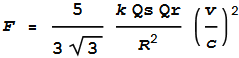--- (3)

The difference between this and the earlier equation (1) is a factor of 5/7.

One reason for preferring positive charge is that it doesn’t move around.  Negative charge comes from excess electrons, which are free to move about and this might negate the benefits.  Also, positive is preferred because excess electrons can more easily leak away or be ejected, discharging the apparatus.  Whereas excess protons can’t leave.

#### Rotating faster than the current

The fact that both negative and positive rings produce a net force in the same direction (although by different amounts) could well be the huge bonus we’re after.

Earlier we rotated our superconducting ring in a direction opposite to the electron current to make the protons orbit instead.  What if we rotated our ring faster than 15cm/s, i.e. faster than what the electrons moved through the ring?

Both protons and electrons would now be orbiting in the same direction, with the protons moving faster.  Both charge types would be contributing to the net force, making it stronger again.  The new net force (adding formulas (1) and (3)) becomes: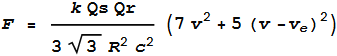--- (4)

Where:
v is the rotational speed of the ring
ve is the velocity of the electrons relative to the ring, in this case 0.147m/s

By rotating the ring at 1m/s the net force becomes 0.00037N.  By rotating at 10m/s it becomes 0.041N.  Rotating at 100m/s gives 4.2N.  And at 300m/s gives 38N.

These are certainly noticeable forces, and actually usable.  38 Newtons can accelerate a 3.8kg mass at 1 G.

The benefit of rotating the superconductor as a whole is that we don’t need to care so much about how fast the current is flowing, because our rotation speed will be much greater.  So we can use cheaper superconducting materials that don’t allow huge currents.  We can probably also use regular conductors (e.g. copper wire electromagnets), although these will need to be kept powered by an electrical supply.

Either way, it is important to keep some kind of current flowing within the ring, otherwise the free electrons might move in a manner that negates the effects, meaning that a neutral spinning disc won’t achieve anything.  For the same reason, it may be necessary to keep a net charge on the sphere.

#### Closing thoughts

If the above is correct then why hasn’t it been noticed before?  Perhaps it has.  There is a fellow by the name of Eugene Podkletnov who claimed to have discovered an ‘antigravity’ effect using a rotating superconducting disc.  There are few details available and, amidst much controversy, counter-claims and denials, it is generally dismissed by the scientific community as erroneous.  But if there’s truth to it, it might be that he was using a method similar to the above without understanding why it worked.

archive.wired.com/wired/archive/6.03/antigravity_pr.html

This snippet is of interest:

We found that any object above the disc lost some of its weight, and we found that if we rotated the disc the effect was increased.”  (emphasis added)

This is in line with what is being described here.  Could it be that the object had a slight charge?  What would happen if they replaced it with something highly-charged?

If anyone has the necessary equipment and materials to try this out, feel free to do so!

 By comparison, Earth-bound vehicles are not limited by ejectable mass but available energy.  An electric car will come to a stop when its batteries are used up.  But a spacecraft without propellant has no control over its trajectory, no matter how much electricity can be drawn from its solar panels.
 The full expression is here.  Replace v with v/c.  It works for any charge signs (+/-).
 The voltage/charge relationship is given by the relationship V = k Q/r, where r is the sphere’s radius – 0.15m in this case.
 http://en.wikipedia.org/wiki/Ultimate_tensile_strength

 Previous Contents Next chapter